# Calculate falling distance when object is already in motion

## Homework Statement

A person looking out of a window of a tall building sees a bucket fly past the window at 30 m/s, then hears the bucket hit the ground 8 seconds later. At what altitude is the observer located? Assume negligible air resistance and speed of sound at 340 m/s.

The problem I am having is that the sound takes an unknown time to arrive at the observer after hitting the ground.

Using the common kinematic equations and substitution, I have a couple of formulas in the link.

What are the steps using my equation, if correct? I haven't managed to do it.

Or, how do I solve it after setting equation#1 equal to velocity * (total time - time for sound to reach observer)?

I have found various equations for solving similar penny/stone in the well problems , but they all assume a starting velocity of zero.

As an additional question, what area of algebra should I practice here, in order to solve equations like this?

## Homework Equations

http://s31.postimg.org/d7moph8wr/well.jpg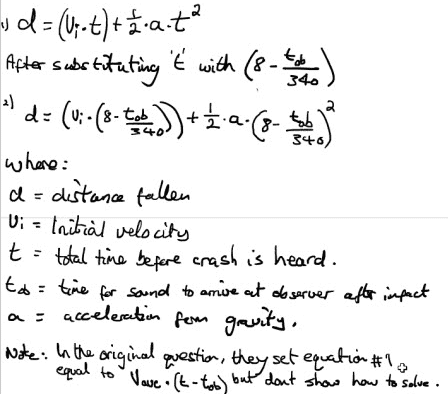[Image inserted by moderator]

## The Attempt at a Solution

The problem I am having is that the sound is already in motion when the time begins, and the sound takes an unknown time to arrive at the observer after hitting the ground due to the unknown distance.

Last edited by a moderator:

gneill
Mentor
Hi califauna, welcome to Physics Forums!

Why don't you write separate equations of motion for the bucket and the sound to begin with? Use different variables for time for each so that both may start from zero. What do you know about the sum of the two times?

Hi, Im not exactly sure what you mean for the motion of the bucket. I think equation number one is an equation for the motion of the bucket until it it hits the ground isnt it (assuming there is no extra time compenent for the sound to arrive at the listeners ears) ? Regarding the time for sound to reach the observer, would it be something like this :

Tob(time to reach observer after hiting ground)=altitude/340

??

Last edited:
by the way, as you may have noticed, the equation I wrote is wrong to start with. I think I should have substituted t (total time to hear sound) for (8-d/340) , not (8-t/340).

gneill
Mentor
Hi, Im not exactly sure what you mean for the motion of the bucket. I think equation number one is an equation for the motion of the bucket until it it hits the ground isnt it (assuming there is no extra time compenent for the sound to arrive at the listeners ears) ? Regarding the time for sound to reach the observer, would it be something like this :

Tob(time to reach observer)=altitude/340

Yes, that's the right idea. Note that the bucket falling and the sound rising both cover the same path (in different directions, of course) so they must cover the same distance equal to the altitude. Call it d.

To begin with you can treat both motions separately in order to establish their equations of motions.

Write out the equations for the each. So for example, for the sound the distance d is given by: d = vs*ts, where vs and ts are the speed of sound and the time that the sound takes in moving from the impact site to the observer's ear.

Do the same for the falling bucket, using a different time variable to represent the time taken for the bucket to pass the observer and finally reach the impact site.

Finally, you have a third equation that tells you what the sum of those two times must be.

haruspex
Homework Helper
Gold Member
Give Califauna time to respond. May be in a different timezone.

Yes, that's the right idea. Note that the bucket falling and the sound rising both cover the same path (in different directions, of course) so they must cover the same distance equal to the altitude. Call it d.

To begin with you can treat both motions separately in order to establish their equations of motions.

Write out the equations for the each. So for example, for the sound the distance d is given by: d = vs*ts, where vs and ts are the speed of sound and the time that the sound takes in moving from the impact site to the observer's ear.

Do the same for the falling bucket, using a different time variable to represent the time taken for the bucket to pass the observer and finally reach the impact site.

Finally, you have a third equation that tells you what the sum of those two times must be.

Like this?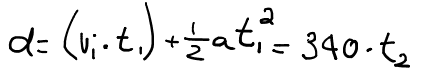gneill
Mentor
That's the idea. Make sure you properly identify which "t" is which. There's no subscript on the t in the squared term you wrote.

What other equation do you have that relates t1 and t2?

•califauna
That's the idea. Make sure you properly identify which "t" is which. There's no subscript on the t in the squared term you wrote.

What other equation do you have that relates t1 and t2?

I changed it

That's the idea. Make sure you properly identify which "t" is which. There's no subscript on the t in the squared term you wrote.

What other equation do you have that relates t1 and t2?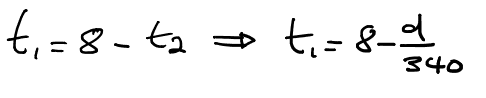gneill
Mentor
Okay. Proceed. Solve for the two times.

•califauna
Okay. Proceed. Solve for the two times.

I cant . I cant get t1 on its own. I can only get this: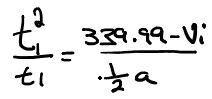gneill
Mentor
Start with the one in post #8. Substitute for either t1 or t2 using the relationship in post #11.

•califauna
Stuck here: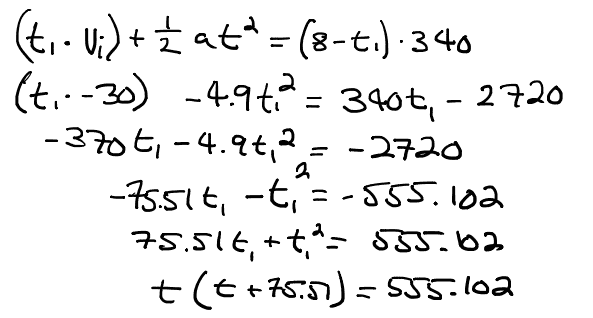gneill
Mentor
You're doing fine. Hint: Quadratic Formula

•califauna
Thanks. Ill get back to this in a few days after going over quadratic formulas again ( and a couple of exams are finished).

Solved it using quadratic formula as suggested. Time to reach observer is 1.251 seconds. Total distance from observer is 425.58 meters.

Thanks again for the help here.